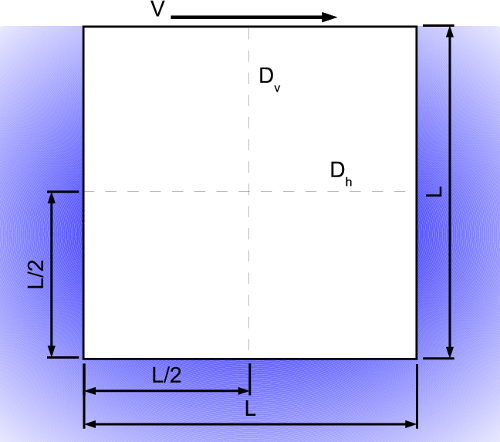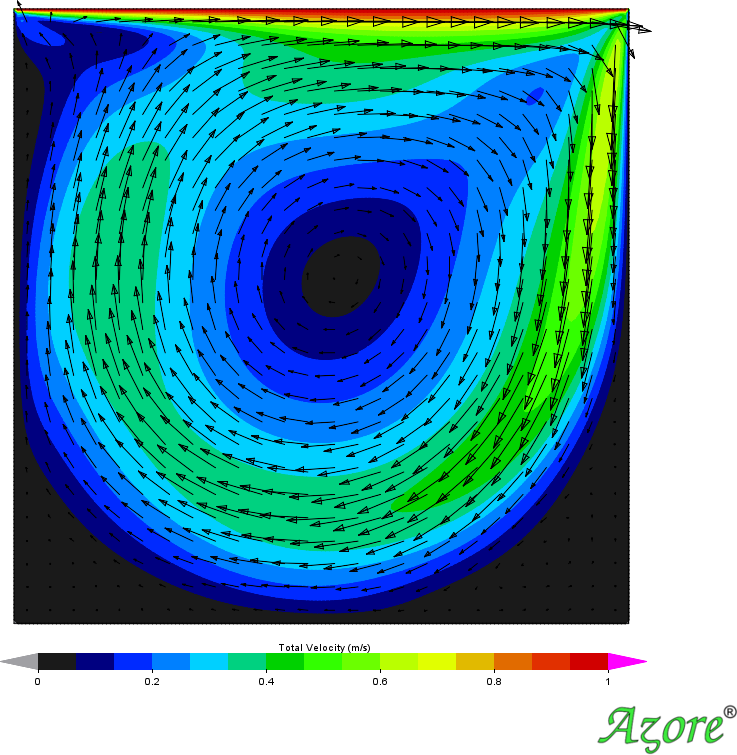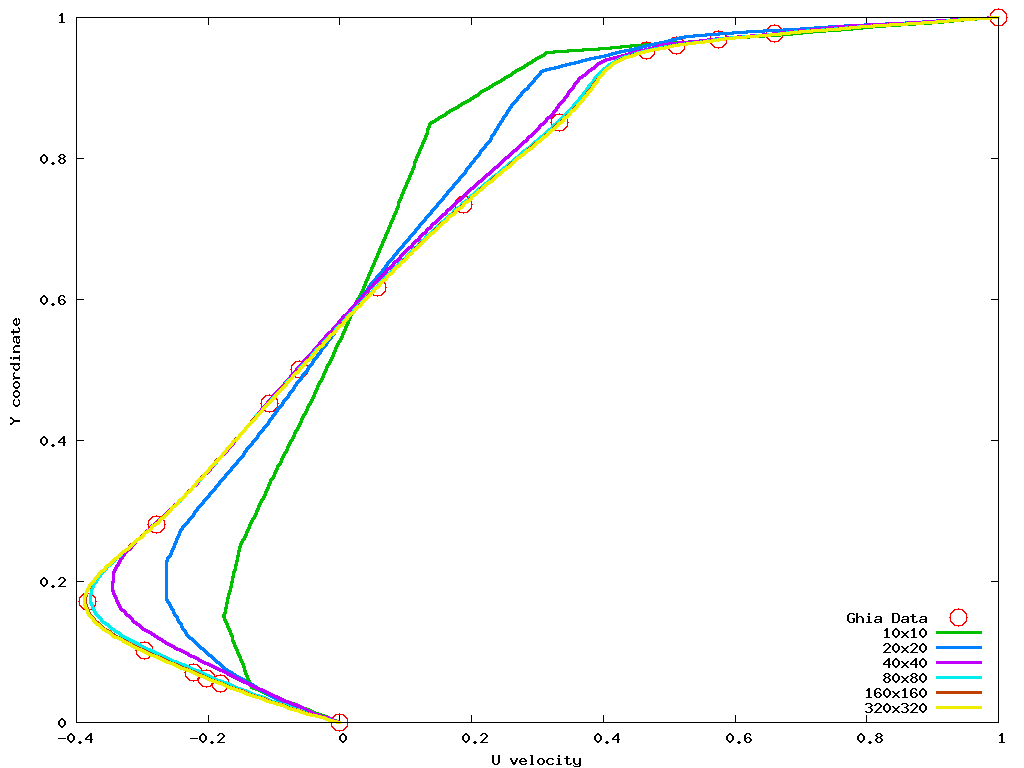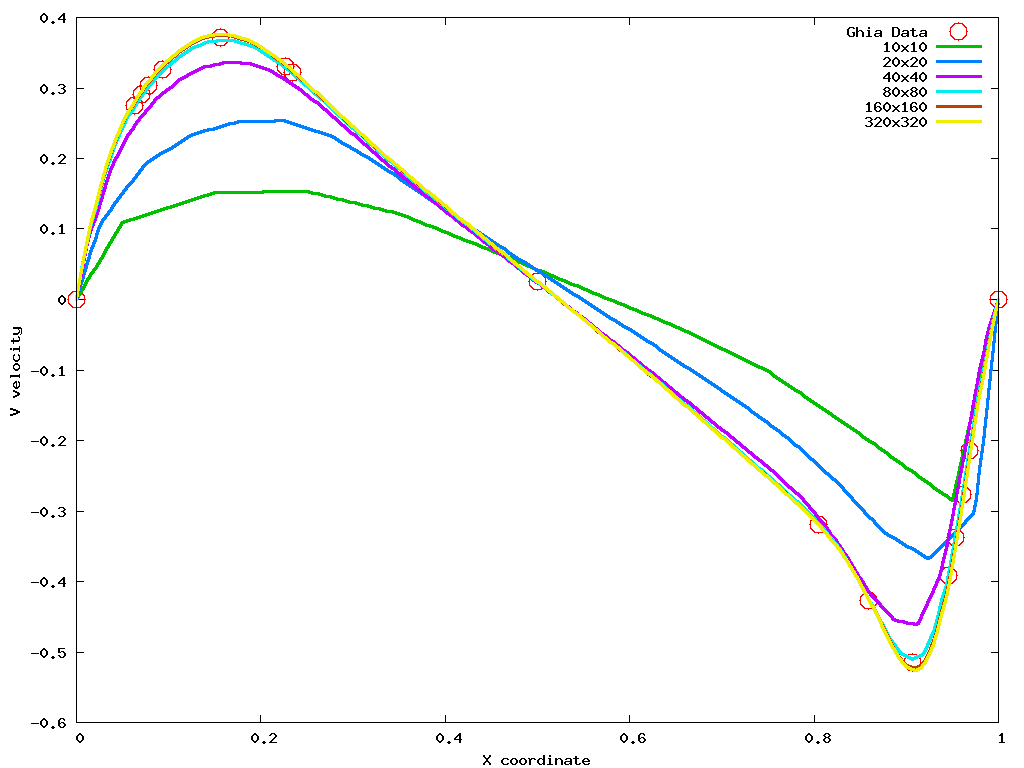### Introduction

The classic two dimensional driven cavity test case is the square driven cavity. The classic literature reference for this case is typically Ghia  who provides tabular solution data for comparison. The fluid motion in the cavity is driven by shear forces due to a moving wall boundary condition at the top of the cavity. Figure 1 details the geometry of the cavity along with the dashed lines Dh and Dv showing where data is taken for comparison.Figure 1

### Model Setup

The Reynolds number of the cavity is used to classify the flow in the square cavity. The fluid density, ρ, viscosity, μ, and size of the cavity, L are used to determine the Reynolds number of the cavity for the cases to be considered here. Ghia tabulates data for several different Reynolds numbers, (100, 400, and 1000). For this study a single Reynolds number is selected (Re =1000) using 1 for ρ, length L, and velocity V and μ=0.001 for viscosity. A systematic grid refinement via six different uniformly spaced square control volume mesh topologies are considered (10x10, 20x20, 40x40, 80x80, 160x160, and 320x320). Figure 2 shows a typical flow field for this problem. The color contours shown in this figure are of the total velocity of the fluid and the vectors indicate the directionality of the fluid motion.Figure 2

### Model Results

Figure 3 shows line graphs of the horizontal velocity predicted by Azore® along the vertical line Dv. There are six lines shown on this graph, one line for each mesh density, along with one set of point data, shown as circles, for the data presented by Ghia. Note that as the mesh density increases the prediction become closer to the data. Figure 4 shows line graphs of the vertical velocity predicted by Azore along the horizontal line Dh. Just as with Figure 3 six lines are shown one for each mesh density. Note these results show a marked differences in the solution predictions as the mesh density increases, especially in the area on the right side of the cavity where the vertical velocity gradients are the largest. These results in general show that a grid independent solution is achieved with the 80x80 mesh density with only small improvements in solution fidelity as the mesh density is doubled to 160x160 and 320x320.Figure 3Figure 4

 U. Ghia, K.N. Ghia, and C.T. Shin, High-Resolution for incompressible flow using the Navier-Stokes equations and a multigrid method, Journal of Computational Physics 48 (1982), 387–411.